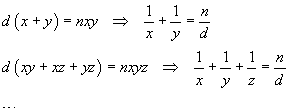Egyptian fractions

(notes by Roberto Bigoni)

versione italiana

Egyptyan fractions calculator
 fraction max. length max. n. sol. time (s)
result

The Javascript application on this page allows you to produce expansions in different sums of unit fractions, that is fractions with the numerator equal to 1 and all with different denominators, of positive fractions less than 1.

These expansions are called Egyptian fractions, because they are used in the famous Rhind papyrus, preserved and exposed to the public at the British Museum in London.

In more recent times, several algorithms for the solution of the problem have been proposed by Fibonacci in his Liber Abaci (1202). Among these algorithms, there is what is today known as the greedy algorithm, a variant of which is implemented by the application.

In general the problem admits several solutions. This application calculates the solutions with the lowest possible number of summands up to a predetermined maximum (6 by default) and up to a predetermined maximum number of solutions (1 by default). For non-trivial fractions and for high values of these two parameters the calculation time can be very long, so you should set the maximum computing time (1s by default) to a value dependent on your patience.

The expansion of a fraction in Egyptian fractions coincides with the search for solutions of special kinds of diophantine equations, that is, those with the following formswhere n and d are known natural numbers, and x, y, z, ... are the unknowns, whose values must also be searched in the set of natural numbers.

last revised: November 2021